# JAC Class 9th Science Solutions Chapter 8 Motion

## JAC Board Class 9th Science Solutions Chapter 8 Motion

JAC Class 9th Science Motion InText Questions and Answers

Page 100

Question 1.
An object has moved through a distance. Can it have zero displacement? If yes, support your answer with an example.
Yes, an object can have zero displacement even after it has moved through a distance. We know that distance is just the length of path an object has covered irrespective of its direction or position with reference to a certain point, whereas the shortest distance measured from the initial to the final position of an object is known as displacement. For example, an object starts from point A and after covering a distance of say 50 metres, reaches at point B. Hereafter, it again moves back to point A.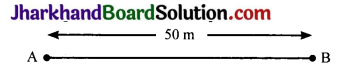Here the distance covered by an object is = AB + BA = 50 m + 50 m = 100 m
whereas displacement of object is = AB – BA = 50 m – 50 m = 0 m.
As initial position of object is same as that of its final position, hence its displacement, which is distance measured from the initial to the final position, is zero.

Question 2.
A farmer moves along the boundary of a square field of side 10 m in 40s. What will be the magnitude of displacement of the farmer at the end of 2 minutes 20 seconds from his initial position?
Given, length of each side = 10 m
Distance covered in 1 lap = Perimeter of ABCD = 4 × 10 = 40 m
Time taken by farmer to cover 1 lap = 40s
Speed of farmer = $$\frac{40}{40 s}$$ = 1m/s
Distance covered by farmer in 2 minutes 20s = Speed × Time = 1 × 140 s = 140 m
Number of laps covered = $$\frac{140}{40}$$
= 3.5 laps
After 140s, the farmer will be at position C (i.e., 3 and $$\frac{1}{2}$$ laps)
Displacement = AC = (AB2 + BC2)1/2
= (100 + 100)1/2
= 10√2 = 10 × 1.414 = 14.14m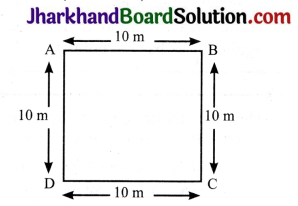Question 3.
Which of the following is true for displacement?
1. It cannot be zero.
2. Its magnitude is greater than the distance travelled by the object.
Both of the statements are not true as:

1. Displacement can be zero.
2. Its magnitude is either less or equal to the distance travelled by the object

Page 102

Question 1.
Distinguish between speed and velocity.

 Speed Velocity (a) The distance travelled by a moving body per unit time is called its speed. (a) The distance travelled by a moving body, in a particular direction, per unit time is called its velocity. (b) It is a scalar quantity. (b) It is a vector quantity. (c) It can be changed only by changing the distance travelled by a body in a particular time. (c) It can be changed by changing the object’s speed, direction of motion or both.

Question 2.
Under what conditions is the magnitude of average velocity of an object equal to its average speed?
The magnitude of average velocity of an object is equal to its average speed only when it is moving in a straight line without turning back.Question 3.
What does the odometer of an automobile measure?
Odometer of an automobile measures the distance covered by the automobile.

Question 4.
What does the path of an object look like when it is in uniform motion?
The path of an object looks like a straight line when it is in uniform motion.

Question 5.
During an experiment, a signal from a spaceship reached the ground station in five minutes. What was the distance of the spaceship from the ground station? The signal travels at the speed of light, that is, 31/2 ,108ms-1
Time taken = 5 minutes = 5 × 60s = 300 seconds
Speed of signal u = 3 × 108 m/s
Distance = ?
Speed = Distance / Time
∴ Distance = speed × time
Distance = 3 × 108 × 300 = 9 × 1010 m

Page 103

Question 1.
When will you say a body is in
(a) uniform acceleration?
(b) non – uniform acceleration?
(a) A body is said to be in uniform acceleration if it travels in a straight line and its velocity increases or decreases by equal amounts in equal intervals of time. Acceleration due to gravity is an example of uniform acceleration.

(b) A body is said to be in non – uniform acceleration if the rate of change of its velocity is not constant, Acceleration of a train running from one station to another is a nonuniform acceleration.

Question 2.
A bus decreases its speed from 80 km h-1 to 60 km h-1 in 5s. Find the acceleration of the bus.
Initial speed of the bus (u) = 80 km h-1
$$\frac{80 \times 1000 \mathrm{~ms}^{-1}}{60 \times 60}$$ = $$\frac{800 \mathrm{~ms}^{-1}}{36}$$
Final speed of the bus (v) = 60 km h-1
= $$\frac{60 \times 1000 \mathrm{~ms}^{-1}}{60 \times 60}$$ = $$\frac{600 \mathrm{~ms}^{-1}}{36}$$
Time in transition (t) = 5s
The acceleration of the bus (a)
$$\frac{\mathrm{v}-\mathrm{u}}{\mathrm{t}}$$ = $$\frac{\left(\frac{800}{36}-\frac{600}{36}\right)}{5}$$ = $$\frac{\left(\frac{-200}{36}\right)}{5}$$
$$\frac{5.55 \mathrm{~ms}^{-1}}{5 \mathrm{~s}}$$ = 1.11 ms-1Question 3.
A train starting from a railway station and moving with uniform acceleration attains a speed 40 km h-1 in 10 minutes. Find its acceleration.
Initial speed of the train (u)
= 0 ms-1
Final speed of the train (v) = 40 km h-1
= $$\frac{40 \times 1000}{60 \times 60} \mathrm{~ms}^{-1}$$ = $$\frac{400}{36} \mathbf{m s}^{-1}$$
Time in transition (t) = 10 minutes = 10 × 60s = 600s
The acceleration of the train (a) = $$\frac{\mathrm{v}-\mathrm{u}}{\mathrm{t}}$$ = $$\frac{\left(\frac{400-0}{36}\right)}{600}$$ = $$\frac{11.11}{600}$$ ms-2
= 0.0185 ms-2

Page-107

Question 1.
What is the nature of the distance-time graphs for uniform and non-uniform motion of an object?
(a) For uniform motion, the distance time graph is a straight line inclined with the time-axis.
(b) For non – uniform motion, the distance – time graph is a curved line.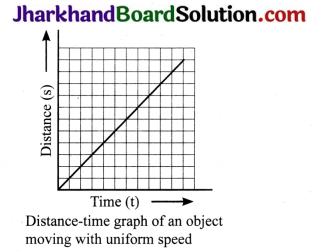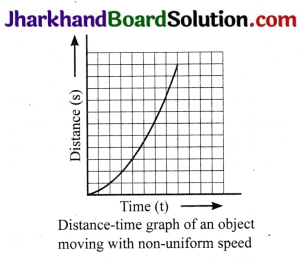Question 2.
What can you say about the motion of an object whose distance-time graph is a straight line parallel to the time axis?
Motion of an object whose distance – time graph is a straight line parallel to the time axis is not moving at all and is in the state of rest.

Question 3.
What can you say about the motion of an object if its speed – time graph is a straight line parallel to the time axis?
The motion of an object, if its speed – time graph is a straight line parallel to the time axis, indicates that the object is moving with a uniform speed.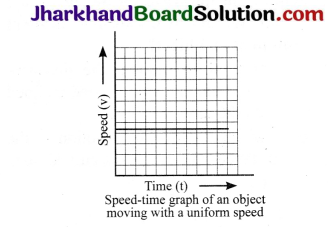Question 4.
What is the quantity which is measured by the area occupied below the velocity – time graph?
Displacement covered by the body in the given time interval.

Page 110

Question 1.
A bus starting from rest moves with a uniform acceleration of 0.1 ms-2 for 2 minutes. Find
(a) the speed acquired,
(b) the distance travelled.
Here,
u = 0, a = 0.1 ms-2, t = 2 min = 120s
(a) v = u + at = 0 + (0.1 × 120) = 12 ms-1
(b) s = ut + $$\frac{1}{2}$$ at2
= (0 × 120) + ($$\frac{1}{2}$$ × 0.1 × (120)2)
= 720m.

Question 2.
A train is travelling at a speed of 90 km h-1. Brakes are applied so as to produce a uniform acceleration of -0.5 ms-2. Find how far the train will go before it is brought to rest.
Here, u = 90 km h-1 = 90 x $$\frac{5}{18}$$ ms-1 = 25 ms-1
a = O.5 ms-2
v= O
As v2 – u2 = 2as
O2 – (25)2 = 2 × (-0.5) × s
or s = $$\frac{-(25 \times 25)}{2 \times-0.5}$$ = $$\frac{-625}{-1}$$ = 265m.

Question 3.
A trolley, while going down an inclined plane, has an acceleration of 2 cm s-2. What will be its velocity 3s after the start?
Initial velocity of trolley (u) = 0 cm s-1
Acceleration (a) = 2 cm s-2
Time (t) = 3s
We know that final velocity (v) = u + at = 0 + (2 × 3) cm s-1
∴ The velocity of train after 3 seconds = 6 cm s-1

Question 4.
A racing car has a uniform acceleration of 4ms-2. What distance will it cover in 10 s after start?
Initial velocity of the car (u) = 0 ms-1
Acceleration (a) = 4ms-2
Time (t) = 10s
We know distance (s) = ut + $$\frac{1}{2}$$ at2
∴ Distance covered by car in 10 s
=0 × 10 + $$\frac{1}{2}$$ × 4 × 102
= 0 + ($$\left(\frac{1}{2} \times 4 \times 10 \times 10\right)$$m
= $$\frac{1}{2}$$ x 400 m = 200m.

Question 5.
A stone is thrown in a vertically upward direction with a velocity of 5 m s-1. If the acceleration of the stone during its motion is 10 m s-2 in the downward direction, what will be the height attained by the stone and how much time will it take to reach there?
Given, initial velocity of the stone (u) = 5ms-1, final velocity (v) = 0ms-1
Downward or negative acceleration (a) = – 10ms-2
We know that 2as = v2 – u2
∴Height attained by the stone (s)
$$\frac{(0)^{2}-(5)^{2}}{2(-10)}$$ = $$\frac{-25}{-20}$$ = 1.25m
Also we know that final velocity (v) = u + at
Also, Time (t) = $$\frac{v-u}{a}$$
Time (t) taken by the stone to attain the height (s) = $$\frac{0-5}{-10}$$ s = 0.5s.

JAC Class 9th Science Motion Textbook Questions and Answers

Question 1.
An athlete completes one round of a circular track of diameter 200 m in 40s. What will be the distance covered and the displacement at the end of 2 minutes 20s?
Diameter of circular track (D) = 200m
Radius of circular track (r) = $$\frac{200}{2}$$ =100m
Time taken by the athlete for one round (t) = 40s
Distance covered by athlete in one round
(s) = 2π r = 2 x $$\frac{22}{7}$$ x 100m
Speed of theathlete (v) = $$=\frac{\text { Dis tan ce }}{\text { Time }}$$
$$\frac{2 \times 2200}{7 \times 40}$$ = $$\frac{4400}{7 \times 40}$$ ms-1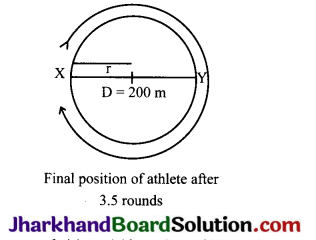∴ Distance covered in 2 minutes 20 seconds (s) or 140s = Speed (s) × Time (t) 4400
= $$\vec{a}$$ × 4 = 2200 m
Number of rounds in 40s = 1 round
Number of rounds in 140s = $$\frac{140}{40}$$ = 3.5
After taking start from position X, the athlete will be at position Y after 3.5 rounds as shown in the figure.
∴ Hence, displacement of the athlete with respect to initial position at X = XY
= Diameter of circular track = 200m.Question 2.
Joseph jogs from one end A to the other end B of a straight 300 m road in 2 minutes 30 seconds and then turns around and jogs 100 m back to point C in another 1 minute. What are Joseph’s average speeds and velocities in jogging: (a) from A to B and (b) from A to C?
(a) From A to B
Time for A → B = 2 min 30 s = (2 x 60) + 30 = 150s Average speed
$$\frac{Total distance}{Time taken}$$ = $$\frac{300}{150}$$ = 2m/s
Average velocity
$$\frac{\text { Displacement }}{\text { Time taken }}$$ = $$\frac{300}{150}$$ = 2m/s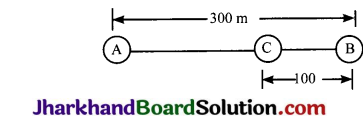(b) From A to C Total distance
= (A to B) + (B to C)
= (300 + 100) = 400m
Time taken= 150 + 60 = 210s
Average speed
$$\frac{Total distance}{Time taken}$$= $$\frac{400}{210}$$ = 1.9m/s
Total displacement = AC
= 300 – 100 = 200m
Average velocity
$$\frac{Displacement}{Time taken}$$ = $$\frac{200}{210}$$ = 0.95 m/s.

Question 3.
Abdul, while driving to school, computes the average speed for his trip to be 20 km h-1. On his return trip along the same route, there is less traffic and the average speed is 40 km h-1. What is the average speed for Abdul’s trip?
Average speed = $$\frac{Total distance}{Time}$$
Suppose the distance from Abdul’s home to school = x km
While driving to school:
Average speed = $$\frac{Distance}{Time}$$
20 km/h = $$\frac{x}{t_{1}}$$…………..(1)
While on his return trip

Average speed =$$\frac{Distance}{Time}$$
40 km/h = $$\frac{x}{t_{2}}$$ ……………(2)
Total distance = x + x = 2x
Total time (t1 + 12)
$$\frac{x}{20}$$ = $$\frac{x}{40}$$ = $$\frac{2 x+x}{40}$$ = $$\frac{3 x}{40}$$
(∴from eq. (1) and (2))
Average speed = $$\frac{2 x}{\left(\frac{3 x}{40}\right)}$$ = $$\frac{2 x \times 40}{3 x}$$
= 26.67 km/h.

Question 4.
A motorboat starting from rest on a lake accelerates in a straight line at a constant rate of 3.0 ms-2 for 8.0s. How far does the boat travel during this time?
Given initial velocity of the motorboat, u = 0 m s-1
Acceleration of the motorboat, a = 3.0 m s-2
Time under consideration, t = 8.0 s
We know that, Distance s = ut + $$\frac{1}{2}$$ at2
∴ The distance travelled by the
motorboat = (0 × 8) + $$\frac{1}{2}$$ × 3.0 × 82
= $$\frac{1}{2}$$ × 3 × 8 × 8m = 96m

Question 5.
A driver of a car travelling at 52 km h-1 applies the brakes and accelerates uniformly in the opposite direction. The car stops in 5s. Another driver going at 3 km h-1 in another car applies his brakes slowly and stops in 10s. On the same graph paper, plot the speed versus time graphs for the two cars. Which of the two cars travelled farther after the brakes were applied?
Initial speed of the first driver
$$=\frac{52 \times 1000}{60 \times 60}$$ =14.44 m/s
Initial speed of the second driver
$$\frac{3 \times 1000}{60 \times 60}$$ = 0.833 m/s
Distance covered by the first driver
$$\frac{1}{2}$$ × 14.44 × 5 = 36.5 m
Distance covered by the second driver
$$\frac{1}{2}$$ × 0.8333 × 10
= 4.165m
Hence, distance travelled by the first car is more.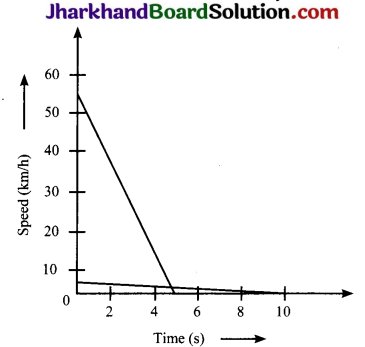Question 6.
Figure shows the distance – time graph of three objects A, B and C. Study the graph and answer the following questions:
(a) Which of the three is travelling the fastest?
(b) Are all three ever at the same point
(c) How far has C travelled when B passes A?
(d) How far has B travelled by the time it passes C?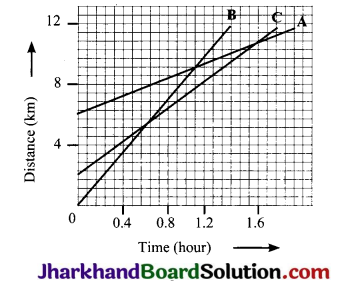(a) B is travelling the fastest.

(b) No. Because three lines do not meet at any point.

(c) When B passes A, C is at a distance of approximately 8 km from the origin.

(d) By the time B passes C, it has travelled 5.5 km.

Question 7.
A ball is gently dropped from a height of 20m. If its velocity increases uniformly at the rate of 10ms-2 with what velocity will it strike the ground? After what time will it strike the ground?
Let us assume that the final velocity with which the ball will strike the ground is V and the time it takes to strike the ground is ‘t’
Initial velocity of the ball, u = 0ms-1
Distance or height of fall, s = 20m
Downward acceleration, a = 10ms-2
As we know, 2as = v2 – u2
v2 = 2as + u2 = (2 × 10 × 20) + 0 = 400ms-1
∴ v = 20ms-1
Final velocity of the ball, v = 20 ms-1
∴ Time taken by the ball to strike,
t =$$\frac{\mathrm{v}-\mathrm{u}}{\mathrm{a}}$$ = $$\frac{20-0}{10}$$ = $$\frac{20}{10}$$ = 2 seconds.Question 8.
The speed – time graph for a car is shown in figure.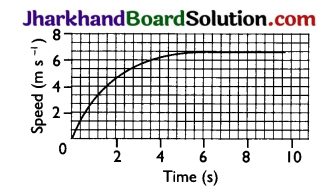(a) Find how far the car travels in the first 4 seconds. Shade the area on the graph that represents the distance travelled by the car during the period.
(b) Which part of the graph represents uniform motion of the car?
(a) In the given graph, 56 full squares and 12 half squares come under the slope for the time of 4 seconds.
Total number of squares = 56 + $$\frac{12}{2}$$ = 62 squares The total area of the squares will give the distance travelled by the car in 4 seconds.
On the time axis, 5 squares
= 2s ∴ 1 square = $$\frac{2}{5}$$s
On the speed axis, 3 squares
= 2m/s ∴ 1 square = $$\frac{2}{3}$$ m/s-1
∴ area of 1 square = $$\frac{2}{3}$$ s x $$\frac{2}{5}$$ms-1
= $$\frac{4}{15}$$
∴ area of 62 squares
$$=\frac{4}{15}$$ m × 62 = $$\frac{248}{15}$$ m = 16.53 m
Therefore, car travels 16.53m in first 4 seconds. The shaded area under speed – time graph represents the distance which the car will travel in the first 4 seconds.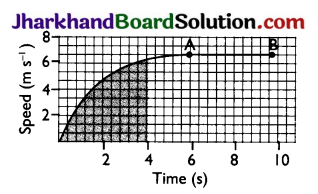(b) The straight line part of graph, from point A to point B, represents uniform motion.

Question 9.
State which of the following situations are possible and give an example for each of these:
1. An obj ect with a constant acceleration but with zero velocity.
2. An object moving with an acceleration but with uniform speed.

1. Possible. Object under free fall due to gravity.
2. Possible. Object moving in circular path.

Question 10.
An artificial satellite is moving in a circular orbit of radius 42250 km. Calculate its speed if it takes 24 hours to revolve around the earth.
Speed of satellite, v = $$\frac{2 \pi \mathrm{r}}{\mathrm{t}}$$
= $$\frac{\left[2 \times \frac{22}{7} \times 42250 \times 1000\right]}{24 \times 60 \times 60}$$
= $$\frac{2 \times 22 \times 42250 \times 1000}{7 \times 24 \times 60 \times 60}$$ms-1